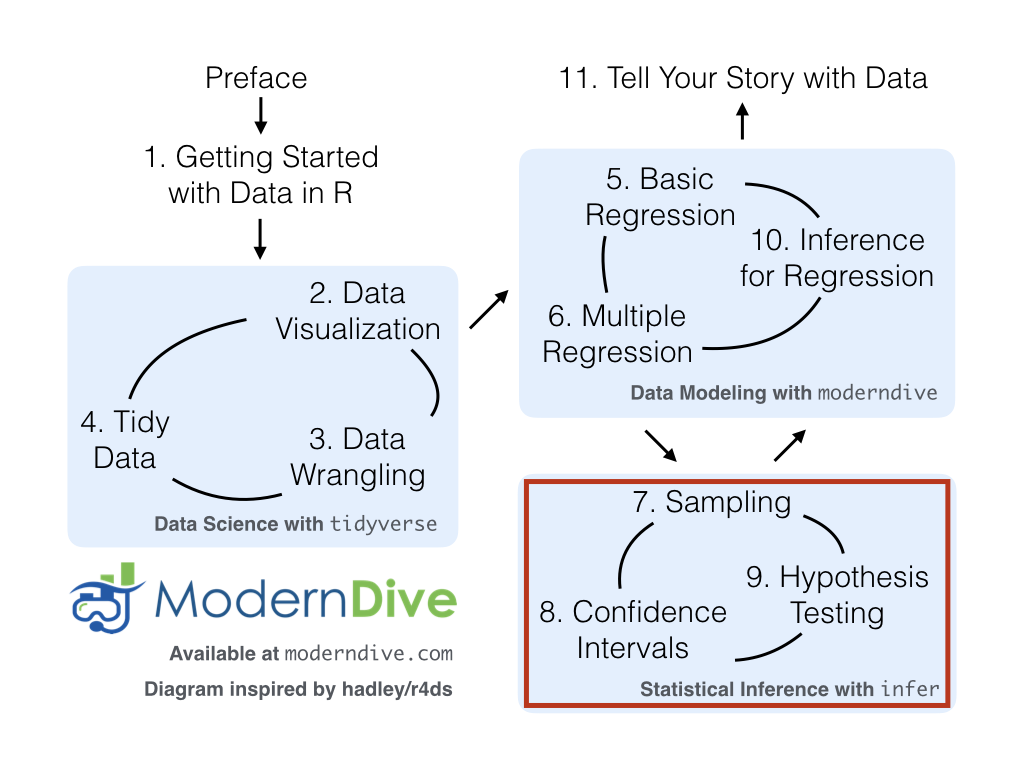## 6.4 Conclusion

An R script file of all R code used in this chapter is available here.

### 6.4.2 What’s to come?

Congratulations! We’ve completed the “Data Modeling with moderndive” portion of this book. We’re ready to proceed to Part III of this book: “Statistical Inference with infer.” Statistical inference is the science of inferring about some unknown quantity using sampling.

The most well-known examples of sampling in practice involve polls. Because asking an entire population about their opinions would be a long and arduous task, pollsters often take a smaller sample that is hopefully representative of the population. Based on the results of this sample, pollsters hope to make claims about the entire population.

Once we’ve covered Chapters 7 on sampling, 8 on confidence intervals, and 9 on hypothesis testing, we’ll revisit the regression models we studied in Chapters 5 and 6 in Chapter 10 on inference for regression. So far, we’ve only studied the estimate column of all our regression tables. The next four chapters focus on what the remaining columns mean: the standard error (std_error), the test statistic, the p_value, and the lower and upper bounds of confidence intervals (lower_ci and upper_ci).

Furthermore in Chapter 10, we’ll revisit the concept of residuals $$y - \widehat{y}$$ and discuss their importance when interpreting the results of a regression model. We’ll perform what is known as a residual analysis of the residual variable of all get_regression_points() outputs. Residual analyses allow you to verify what are known as the conditions for inference for regression. On to Chapter 7 on sampling in Part III as shown in Figure 6.12!FIGURE 6.12: ModernDive flowchart - on to Part III!